[BACK]
 Intelligent Automation & Soft ComputingDOI:10.32604/iasc.2022.027710Article

Spatio-temporal Model Combining VMD and AM for Wind Speed Prediction

1Nanjing University of Information Science and Technology, School of Computer and Software, Nanjing, 210044, China
2IT Fundamentals and Education Technologies Applications, University of Information Technology and Management in Rzeszow, Rzeszow, Voivodeship, 100031, Poland
*Corresponding Author: Yingnan Zhao. Email: zh_yingnan@126.com
Received: 24 January 2022; Accepted: 13 March 2022

Abstract: This paper proposes a spatio-temporal model (VCGA) based on variational mode decomposition (VMD) and attention mechanism. The proposed prediction model combines a squeeze-and-excitation network to extract spatial features and a gated recurrent unit to capture temporal dependencies. Primarily, the VMD can reduce the instability of the original wind speed data and the attention mechanism functions to strengthen the impact of important information. In addition, the VMD and attention mechanism act to avoid a decline in prediction accuracy. Finally, the VCGA trains the decomposition result and derives the final results after merging the prediction result of each component. Contrasting experiments for short-term prediction on the actual wind power dataset prove that VCGA is superior to prior algorithms.

Keywords: Wind speed prediction; gated recurrent unit; squeeze-and-excitation networks; variational mode decomposition; attention mechanism

1  Introduction

Currently, there is a growing need to utilize renewable energy to solve future energy shortages. Thus, new energy systems are replacing many traditional power generation systems. As one of the most potential, abundant, and environmentally renewable resources, wind energy has gained enormous attention from governments and enterprises worldwide [1,2].

The accurate prediction of short-term wind speed is essential to the operation and control of wind power systems. It aids the appropriate sitting of wind power grid connection, reduces voltage and frequency fluctuation caused by wind power variation, and improves the reliability of power grid operation . Typically, wind speed prediction technology is categorized into physical, statistical, and artificial intelligence. The representative approach of the physical model is the Numerical Weather Prediction model (NWP) , which employs real-time meteorological data to generate forecasts. Yet the mathematically rigorous nature of these NWP models makes them suitable for long-term wind speed prediction in specific regions, i.e., it is not ideal for short-term and ultra-short-term wind speed prediction. Meanwhile, the statistical method constructs the nonlinear mapping relationship between historical wind speed data and learns the law of the time series prediction. The main statistical methods comprise the autoregressive moving average model (ARMA) , support vector machines (SVM) , extreme learning machine (ELM) , etc. The basis of the artificial intelligence model is machine learning techniques. An enormous wind speed data describes the complex nonlinear relationship between system input and output. With the rapid development of deep learning techniques, this domain of artificial intelligence is rapidly gaining grounds in its application to short-term wind speed prediction, typical of which is convolutional neural networks (CNN) , back propagation (BP) neural networks , recurrent neural networks (RNN) , gated recurrent unit (GRU) , and long short-term memory (LSTM) . These methods combine existing wind speed prediction technology with a hybrid neural network model to get excellent results.

However, due to wind power’s intermittency, volatility, and uncertain nature, the aforementioned methods are usually combined with specific processing methods to obtain relatively stable subsequences in practical applications. The variational mode decomposition (VMD) method  is a good choice, which has better noise robustness, and the number of components is much smaller than empirical mode decomposition (EMD)  and ensemble empirical mode decomposition (EEMD)  through the reasonable control of convergence conditions. Moreover, when the input time series is long, LSTM, GRU, and other networks are prone to lose sequence information. Therefore, it is challenging to deal with the structural information between data affecting the model’s accuracy . To solve this problem, the attention mechanism , which can assign different weights to the input features so that the features containing important information will not disappear with the increase in step size, is adopted. Embedding the attention mechanism in the deep neural architecture, the model can make learning the long-distance interdependent relationship in sequences easier and highlight the influence of more critical information.

In recent years, there have been remarkable achievements in wind speed prediction. Beyond the usual temporal correlation, spatial correlations, an essential feature of wind speed, have gained considerable research attention. Consequently, spatial and temporal correlation analysis has become a research hotspot . These algorithms consider data’s temporal and spatial characteristics to improve the prediction accuracy. Therefore, this paper proposes a spatio-temporal model (VCGA) enhanced by the attention mechanism and VMD.

The main contributions of this paper are as follows.

(1)   Employing the VMD method to process the wind speed data. Consequently, the unstable wind speed sequence is transformed into a relatively stable subsequence to improve the wind speed prediction accuracy.

(2)   Given the irrelevant features in the data that will lead to the decline of model performance, it is necessary to redistribute the feature weights and improve the model performance through the attention mechanism.

(3)   The underlying architecture of VCGA is composed of CNN and GRU. This model can deal with temporal and spatial characteristics of wind speed and employ spatio-temporal correlations in wind speed prediction, enhancing the prediction accuracy.

The remainder of the paper is organized as follows. Section 2 gives the basic principles of VMD and the attention mechanism and background theories about CNN and GRU; Section 3 introduces the spatio-temporal data model of wind speed used in VCGA, the hybrid deep learning framework, and how to integrate the attention mechanism in this framework skillfully. Section 4 is the experimental part, comparing and analyzing with relevant algorithms, proving the superiority of the new algorithm. Finally, Section 5 summarizes the whole paper and points out the direction of further research.

2  Research Methods

2.1 Variational Mode Decomposition

In 2004, K. Dragomiretskiy and D. Zosso proposed VMD, an adaptive, quasi-orthogonal, and completely non-recursive decomposition method . This method is based on classical Wiener filtering, Hilbert transform, and frequency mixing. VMD supposes that each mode has a different central frequency and bandwidth, minimizing the sum of the estimated bandwidths of each modal, thus transforming modal estimation into a variational problem.

To solve this variational problem, the alternate direction method of multipliers  is adopted to obtain all the modes of signal decomposition through iterative updating. The modes containing the main signal and the noise are among the decomposed modes. The mode containing the main signal is reconstructed to achieve the effect of denoising. Therefore, VMD can decompose the original wind speed sequence with nonlinearity and randomness into a series of stable modal components to make the prediction result more accurate.

The essence of VMD is a variational problem, which mainly includes the construction and solution of the variational problem, and its process is as follows.

(1)   First, we need to construct the variational problem. Assuming that decomposing the original signal F into K components, each mode has a limited bandwidth of a central frequency and minimizes the sum of the estimated bandwidths of each modal. The preprocessed space-time data of wind speed is X~(t) , where t represents a particular moment. The specific model is as follows:

min{uk},{ωk}{k=1Kt[(δ(t)+jπt)uk]ejωkt22}

s.t.k=1Kuk=X~(t) (1)

In Eq. (1), K is the number of modes to be decomposed (K is a positive integer), {uk} and {ωk} correspond to the Kth mode component and center frequency after decomposition, respectively, δ(t) is a Dirac function and * is a convolution operation.

(2)   Therefore, to solve Eq. (1), the Lagrange multiplication operator λ is introduced to transform the constrained problem into a non-constrained problem and obtain the augmented Lagrange expression

L({uk},{ωk},{λ})=αk=1K t[ (δ(t)+jπt)uk ]ejωkt 22+ f(t)k=1Kuk(t) 22+ λ(t)f(t)k=1Kuk(t) (2)

In Eq. (2), α is the penalty factor, which is used to reduce the influence of Gaussian noise.

(3)   The parameters {uk}, {ωk}, and λ are iteratively updated by the alternate direction method of the multiplier. The formula is as follows:

u^kn+1=f^(ω)ikui^(ω)+λ^(ω)21+2α(ωωk)2 (3)

ωkn+1=0ω|u^k(ω)|2dω0|u^kn+1(ω)|2dω (4)

λ^n+1(ω)=λ^n(ω)+γ(f^(ω)ku^kn+1(ω)) (5)

In Eqs. (3)(5), f^(ω),ui^(ω),λ^(ω) , and u^kn+1(ω) represent the Fourier transforms of f(ω),ui(ω),λ(ω), and ukn+1(ω) ; n is the number of iterations; And γ is the noise tolerance, which meets the requirement of fidelity of signal decomposition.

(4)   Finally, K decomposed IMF components, denoted as IMFk, can be obtained. Fig. 1 demonstrates the IMF components of the wind speed subseries at different frequencies. The main parameters are set as follows: K  =  8, α  =  2000, γ  =  0, e  =  1e − 7. In Fig. 1, the top is the actual wind speed, followed by IMF0–IMF7, a total of eight components. Fig. 2 shows each spectrum of the corresponding component.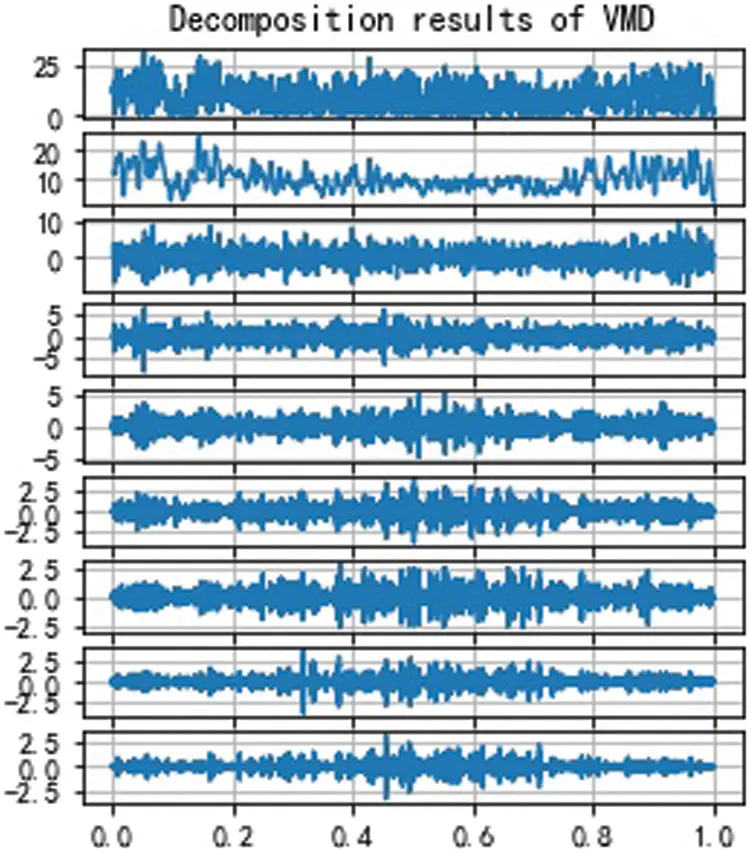Figure 1: IMF components after VMD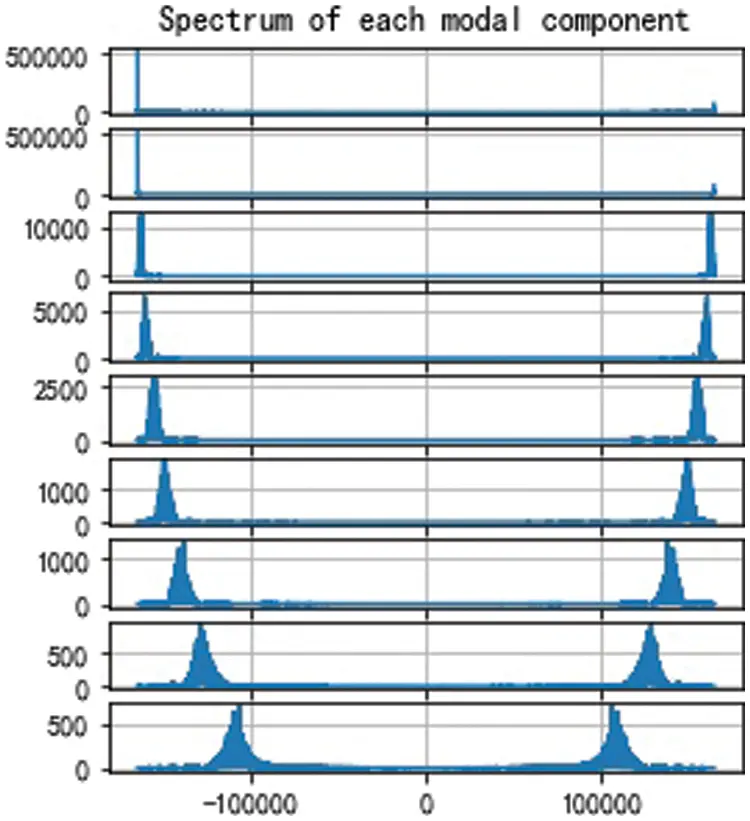Figure 2: Spectrum of components

2.2 Convolutional Neural Networks

CNN adopts the method of local connection and weight sharing to process the original data at a higher and more abstract level, which effectively and automatically extracts the internal features of data . CNN comprises a convolution layer, pooling layer, and full connection layer, which employs the convolution kernels, local pooling operations, and fully connected operations to alternately apply the results of forward and backpropagations to the input data to extract spatial features. The more convolution kernels, the more abstract the extracted features . The model uses the convolution and pooling layers (method may include maximum pooling, average pooling, etc.) to obtain adequate information and automatically extracts feature vectors from data, thus reducing the complexity of feature extraction and data reconstruction and improving the quality of data features. However, it is difficult for CNN to learn the relationship between time-series data prediction. Therefore, it is necessary to strengthen it with the RNN series of typical methods.

2.3 Gated Recurrent Unit

LSTM and GRU are both variants of the RNN [24,25]. LSTM has the function for capturing long-term dependency and is suitable for analyzing time-series data. Still, its complex internal structure, primarily responsible for its long training time, is a considerable disadvantage. Therefore, GRU is used to optimize and improve LSTM, thus reducing training parameters and ensuring prediction accuracy . In addition, GRU contains the update gate and the reset gate. Therefore, compared with LSTM, GRU has fewer structural parameters and faster convergence speed. Fig. 3 shows the structure of GRU.Figure 3: The structure of gated recurrent unit (GRU)

In Fig. 3, × is the scalar multiplication of the matrix, and 1 − indicates that the data transmitted forward by the link is 1  −  zt. zt and rt represent the update gate and reset gate, respectively. xt is the input and ht is the output of the hidden layer. σ is the activation function Sigmoid and tanh is the activation function Tanh. Therefore, the activation functions σ and tanh are defined by Eqs. (6) and (7).

σ=11+ex (6)

tanh=exexex+ex (7)

Eqs. (8)(11) are for the derivation of the output of the hidden layer ht.

zt=σ(Wz[ht1,xt]) (8)

rt=σ(Wr[ht1,xt]) (9)

h~t=tanh(W[rtht1,xt]) (10)

ht=(1zt)ht1+zth~t (11)

where x = [x1, x2, ⋅ ⋅ ⋅ ⋅ ⋅ , xt] is the output of the fully connected layer, h~t is the input to the reset gate, which is the combination of the previous moment state ht−1 and the current input xt, and W is the weight matrix.

2.4 Attention Mechanism

The attention mechanism [27,28] simulates the human brain’s attention resource allocation mechanism. The human brain, at any instance, is preoccupied with the need to fixate on an area to reduce or ignore distractions from other sites. By doing so, the relevant information within a region is enlarged. Consequently, the attention mechanism functions by concentrating on crucial details to extract more important features through probability distribution, improving the model’s accuracy.

Fig. 4 shows the structure of the attention mechanism. In Fig. 4, xn represents the input of the network, hn corresponds to the output of the hidden layer generated by each input through the network, and αt represents the probability distribution value of the attention mechanism for the output of the hidden layer. y is the output value of the network which combines the attention mechanism.Figure 4: The structure of the attention mechanism

The attention mechanism is typically used in RNN architectures to improve the model performance and has contributed massively to time series prediction. For example, the research in [29,30] proposed attention blocks used in LSTM architectures for time series forecasting. In addition, some researchers combined the attention blocks with CNN architectures for image classification and time-series data .

3  VCGA Algorithm Description

First, the VCGA algorithm would preprocess the original data, i.e., to acquire the original spatio-temporal wind speed series of the target site. Second, this algorithm applies the VMD method to decompose it and gets the IMF components for processed data. Then, the attention mechanism is employed to improve the CNN-GRU network. Finally, the predicted values of each IMF component are acquired and superimposed on the predicted values to obtain the final value through this new network.

Two functional modules make up the new hybrid network model. The two modules introduce the attention mechanism to optimize the performance. One module takes the CNN model as the core and blends in an SE block, whose purpose is to extract the spatial characteristics of wind speed. The other module selects the GRU model to capture temporal dependency and adds an attention layer to avoid information loss in time series. Fig. 5 shows the flow chart of the VCGA algorithm.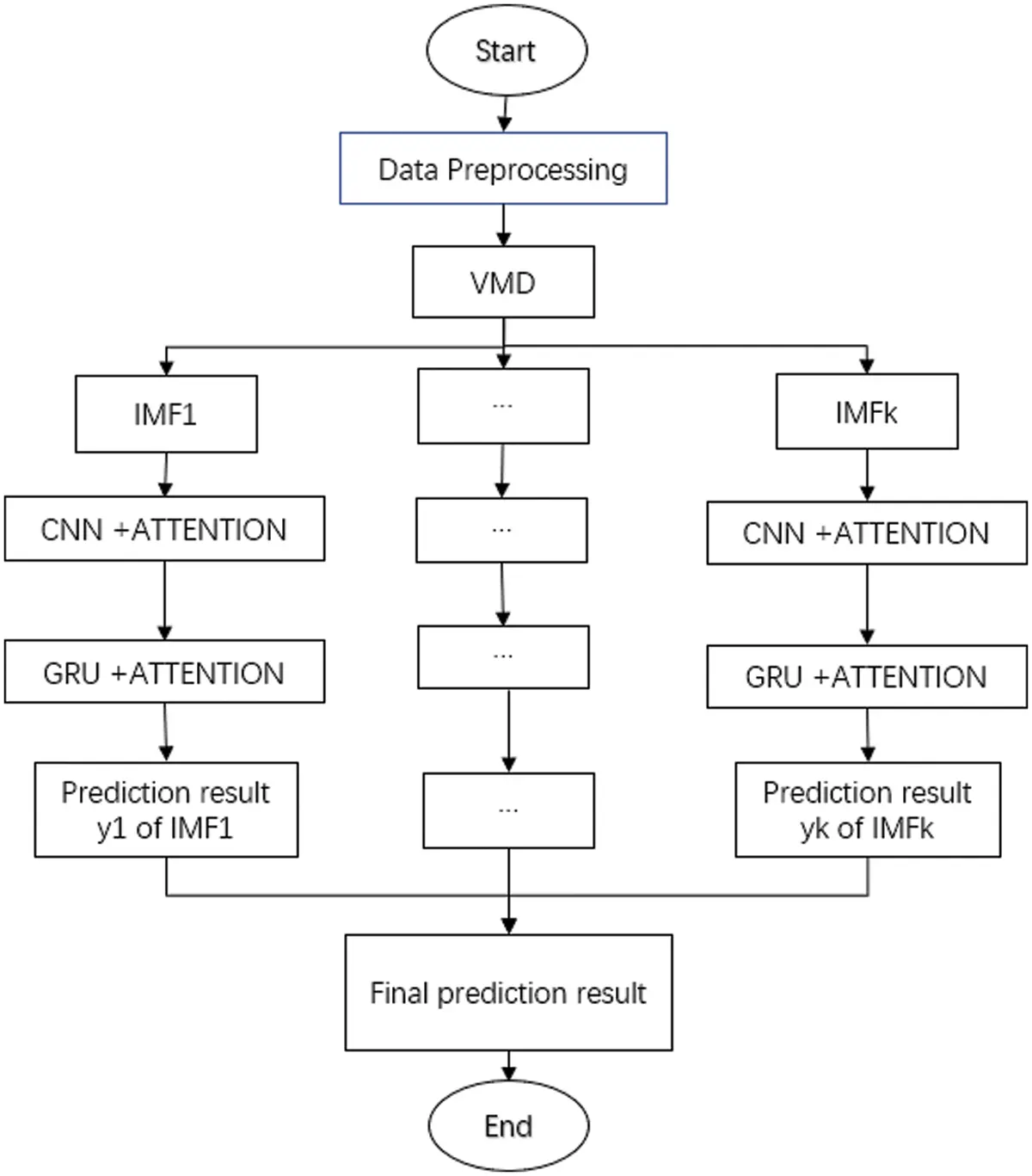Figure 5: Flow chart of VCGA algorithm

3.1 Spatio-temporal Data Model of Wind Speed

Wind speed data usually have both temporal correlation and spatial correlations. For one, the temporal correlation of wind speed data implies that the wind speed at a given location is related to temporal variation. Conversely, the spatial correlation indicates that the wind speed at different areas within a specific geographical scale is not independent of statistics. Even though the wind speed at other sites is different, wind speed data’s temporal and spatial functions are continuous . The above properties are essential references for the better handling of wind speed data.

One difficulty is retaining the spatio-temporal correlation of data without increasing the amount of data. Dimension reduction is an efficient solution . In this paper, a two-dimensional (2D) matrix named spatial wind speed matrix (SWSM) was proposed and spatio-temporal wind speed data were placed in this matrix . Suppose the research object is an array of M rows and N columns on a spatial region that an M  ×  N grid can represent. In this array, we can index each site by a 2D rectangular coordinate (i, j) (1 ≤ i ≤ M, 1 ≤ j ≤ N) and define it as x(i, j)t ∈ RM×N(1 ≤ t ≤ T), where i and j denote the row and column index, respectively, and for each site, the wind speed is a one-dimension (1D) time series. Then, an SWSM x(i, j)t ∈ RM×N can be defined as

xt=[x(1,1)tx(1,2)tx(1,N)tx(2,1)tx(2,2)tx(2,N)tx(M,1)tx(M,1)tx(M,N)t] (12)

Suppose that Fig. 6a is the time-domain wind speed data of site (M, N), and the process of transforming wind speed sequence into the matrix is shown in Fig. 6b.Figure 6: (a) The wind speed time series at the site (M, N) (b) The spatio-temporal data model of wind speed

This matrix approach is referred to and subsequently improved by VMD. Meanwhile, assuming that the time window length is T and the IMF component number is K.

If at a time t, we can denote the value of the site (i, j) for an IMFk component as x(i,j)kt , x(i,j)ktRM×N , so the values of this component IMF at all stations at time t are as follows.

xkt=[x(1,1)ktx(1,2)ktx(1,N)ktx(2,1)ktx(2,2)ktx(2,N)ktx(M,1)ktx(M,2)ktx(M,N)kt] (13)

It is important to note that a single SWSM does not involve any time information because all its elements are observed simultaneously in a single SWSM. Therefore, by organizing SWSMs in chronological order, we can construct a spatio-temporal sequence describing the array’s wind speed. As shown in Fig. 7, we decomposed the wind speed into K components IMF at time t, and different IMF were represented by IMFkt,(i,j) .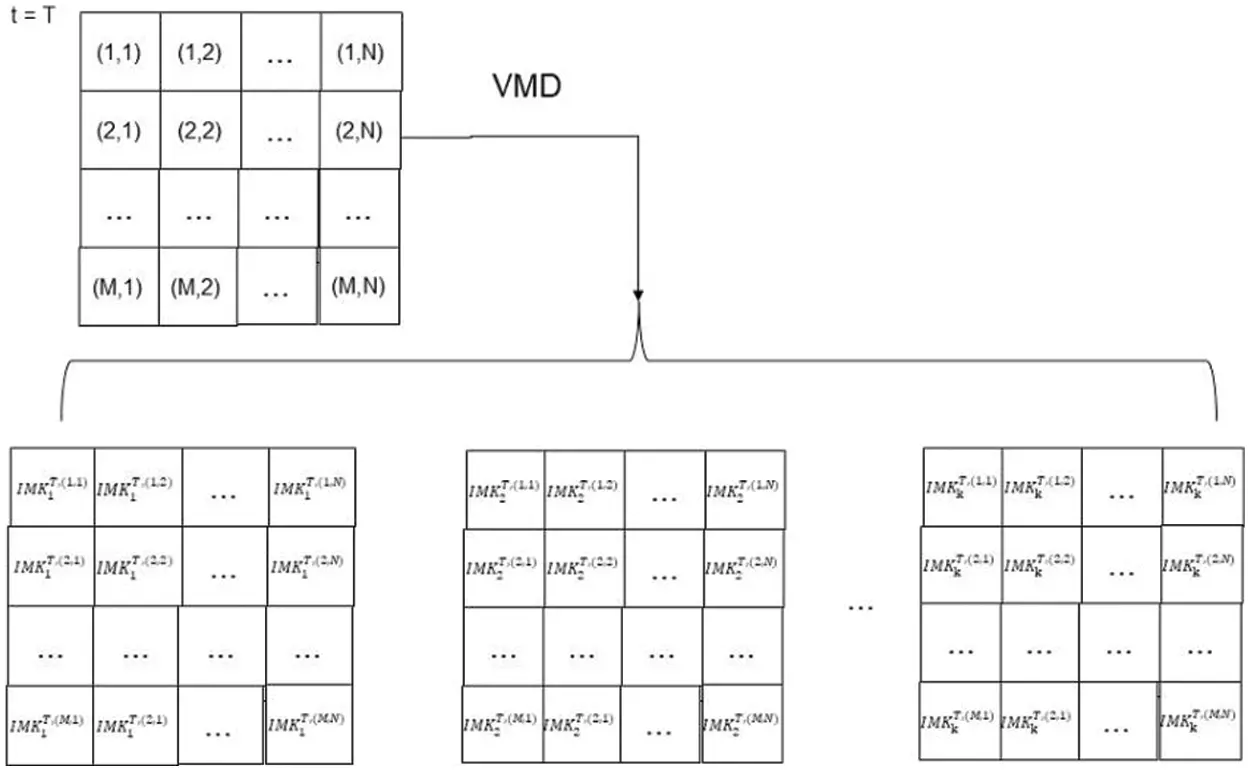Figure 7: Spatio-temporal data model of wind speed after VMD at time T

3.2 Model Architecture

By analyzing the SWSM, the wind prediction model needs a spatial model to extract spatial features and a time model to obtain temporal correlation. Combined with the improvement of previous models, we propose the VCGA model to make a more accurate prediction. The model is mainly divided into the input, SENet, GRU, Attention, and output layers.

The input data of the input layer is the spatial data of the IMF component obtained by VMD decomposition. Then squeeze-and-excitation networks (SENet) , as the underlying structure, receives these data. SENet is the organic combination of CNN and attention mechanism, which can significantly improve the performance of CNN. It employs the channel attention mechanism to automatically learn the importance of different channels to recalibrate channel-wise features, including extracting task-related features and suppressing task-irrelevant features. Another point is that this operation is generic, which means it can be applied into existing network architectures. The SENet layer can make full use of the spatial characteristics of IMF components, receive the 2D tensor of IMF, design convolution operations to increase its depth, and compress the number of parameters. Then, it can carry out feature dimensionality reduction through pooling layer processing, and finally transform the 2D tensor of IMF into a one-dimensional structure by the full connection layer and extract spatial features by layers. The output is denoted as HS =  [HS1, HS2, …, HSi], and the length of the SENet layer is i.

Here is how to get HS by SENet layer.

uc=vcX=s=1CvCsXs (14)

zc=1W×Hi=1Wj=1Huc(i,j) (15)

s=σ(g(z,W))=σ(W2δ(W1z)) (16)

HS=scuc (17)

In Eq. (14), vc represents the c-th convolution kernel, X is the input, and * stands for convolution operation. Eq. (15) is a global average pooling. In Eq. (16), W1z is an operation of full connection layer and the dimension of W1 is Cr  C . Multiplying by W2 is also a full-connection process, and the dimension of W2 is CCr . Thus, the output dimension is 1 * 1 * C, δ is the ReLU function, and δ is the Sigmoid function. In Eq. (17), sc is the weight reflecting the importance of each feature channel, and the weight coefficients of each channel can be learned through sc  ⋅ uc.

In the VCGA algorithm, GRU is adopted as the superstructure of a hybrid deep learning framework to receive spatial features extracted from SENet. A single-layer GRU structure was constructed to fully learn the proposed features to capture their internal variation rules. Simultaneously, the Dropout method  is used to suppress overfitting. h is the output of the GRU layer and the output at step t is expressed as

ht=GRU(HS,t1,HS,t),t[1,i] (18)

The input of the Attention layer is the output vector ht, which is activated by the GRU network layer. The optimal weight parameter matrix is constantly updated, iterated, and calculates the corresponding probabilities of different feature vectors according to the weight allocation principle. The calculation formula of the weight coefficient of the attention mechanism layer can be expressed as

et=utanh(wht+b) (19)

at=exp(et)j=1tej (20)

st=t=1iatht (21)

In Eqs. (19)(21), et represents the attention probability distribution value determined by the output vector ht of GRU network layer at time t; u and w are weight coefficients; b is the bias coefficient; the output of the Attention layer at time t is represented by st.

Finally, the input of the output layer is the output of the Attention layer. Then, the output Y = [y1, y2, ⋅ ⋅ ⋅ ⋅ ⋅, ym] T with the prediction step of m calculated through the full connection layer and the prediction formula can be expressed as

yt=Sigmoid(wost+bo) (22)

In Eq. (22), yt represents the predicted output value at time t; wo is the weight matrix; bo is the deviation vector, and the Sigmoid function was selected as the activation function of the Dense layer.

3.3 Loss Function

The VCGA model selects the Adam (adaptive moment estimation)  optimization algorithm to optimize the model parameters. The algorithm can update the weight of the neural network iteratively based on training data so that the output value of the loss function can reach the optimal value. The loss function of the VCGA model uses the mean square error function (MSE); (23) expresses the formula of MSE.

Loss=1ni=1n(yiy¯i)2 (23)

In Eq. (23), n is the number of samples; yi is the actual value of; and y¯i is the model prediction value.

4  Case Study

4.1 Data Set

The dataset used in this paper is from the Wind Integration National Dataset provided by the National Renewable Energy Laboratory. We collected the selected wind speed data at an interval of 5 min for a 10 × 10 wind turbine array in a wind farm in Wyoming, USA, measured in 2012. Then, we reset the time interval to 10 min for prediction. There are 52560 data in the dataset, among which the highest wind speed is 35.48 m/s and the lowest wind speed is 0.01 m/s. The training set, validation set, and test set are the first 60%, the following 10%, and the last 30% of the data, respectively.

In the experiment, the GPU server is configured as NVIDIA GeForce RTX 2080 Ti, 11G video memory, 11G E5–2678, 24-core CPU, 440GB SSD, and 4TB hard disk. The development environment combines frameworks, including tensorflow2.4, keras2.4.2, and python 3.7. Tab. 1 shows the configuration of the hybrid deep learning framework.4.2 Evaluation Indices

For wind speed prediction, we choose root mean square error (RMSE)  and mean absolute percentage error (MAPE)  as evaluation indexes. MAPE can measure the quality of model prediction results, while RMSE can evaluate the prediction accuracy. The greater the error between the predicted and actual values, the greater the RMSE. Compared with RMSE, MAPE normalized the error of each point and reduced the influence of absolute error brought by some outlier points; the greater the error, the greater the value of MAPE. This is defined explicitly by the following formula.

RMSE=i=1n(yiy¯i)2n (24)

MAPE=100ni=1n|yiy¯iyi| (25)

In Eqs. (24) and (25), n is the number of samples, y¯ is the predicted value, and yi is the actual value.

4.3 Baseline Algorithms

To verify the superiority of the proposed VCGA, we compared the VCGA algorithm with similar algorithms for processing spatio-temporal data, including the CNN-GRU algorithm, VMD-CNN-GRU algorithm (VCG) and CNN-GRU-Attention algorithm (CGA) . Meanwhile, we compare the spatio-temporal algorithms with the temporal algorithms, such as LSTM and GRU . Here the activation function of GRU is ReLU , and the activation function of LSTM is tanh. The time intervals are 20, 30, 60, and 120 min. All algorithms run in the same experimental environment. For each algorithm, experiments test different hyperparameters to determine the best setting for each hyperparameter.

Tabs. 2 and 3 show RMSE and MAPE’s comparison of prediction errors of different algorithms, respectively.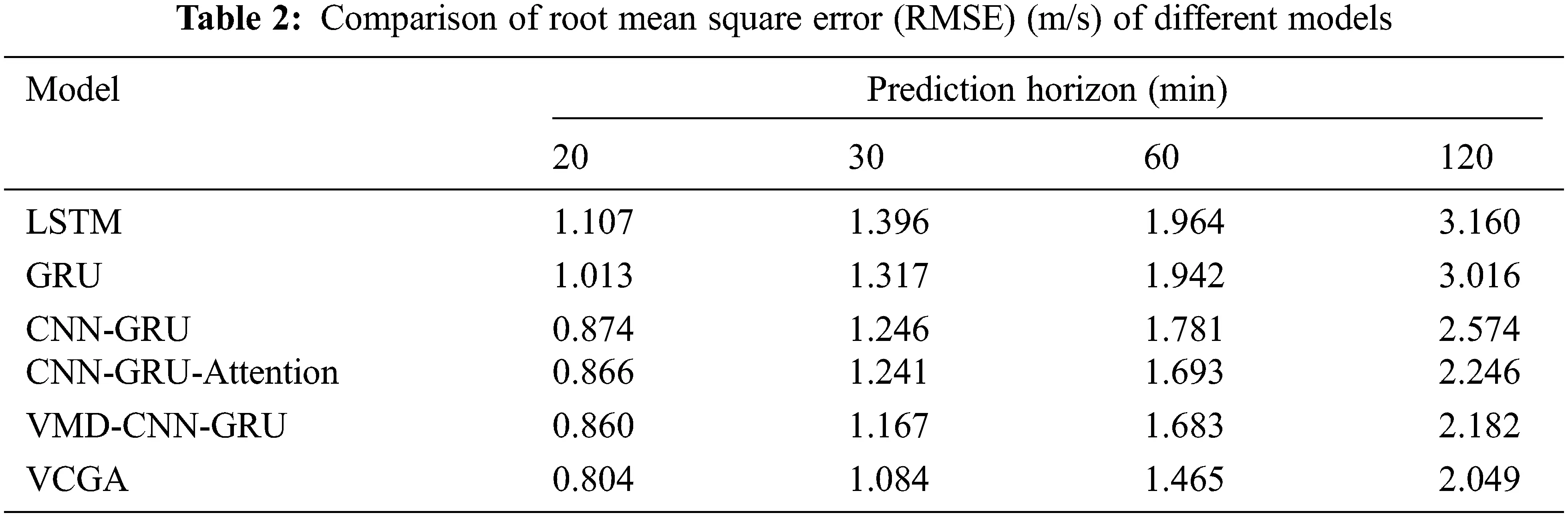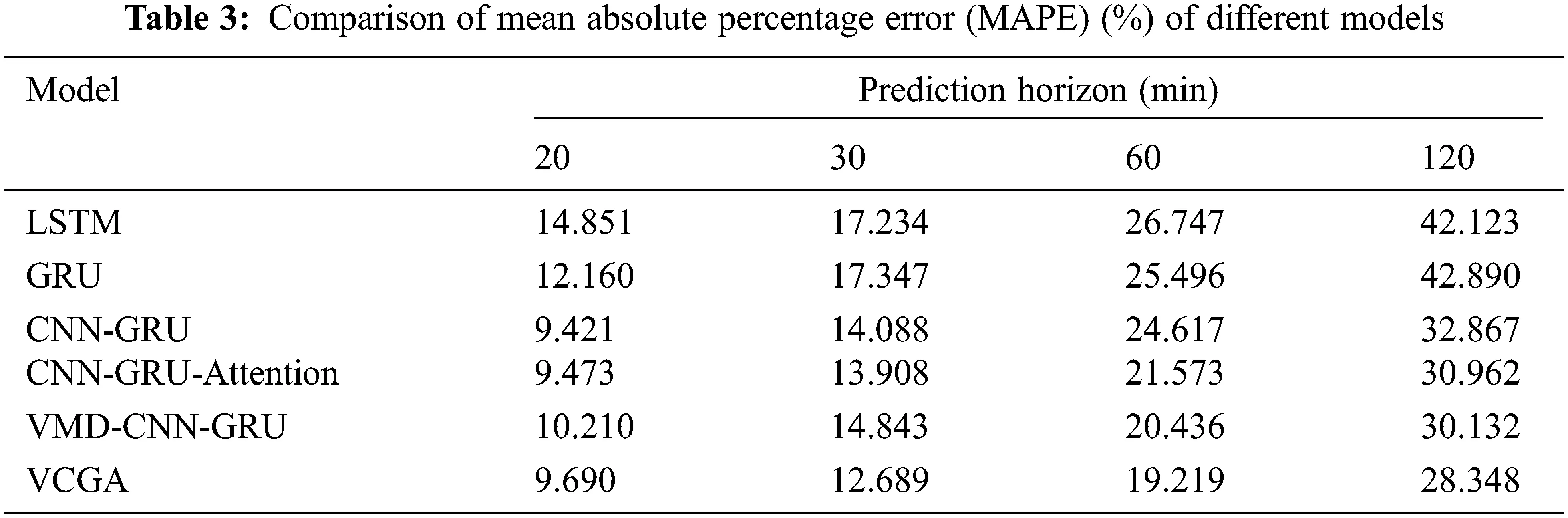First, the temporal algorithms LSTM and GRU are compared. For time intervals of 20, 30, 60, and 120 min, the RMSE of GRU is 8%, 6%, 2%, and 5% lower than LSTM, respectively, with an average decrease of 5.25%. When the prediction time interval is 20 and 60 min, the MAPE of GRU is lower than LSTM by 28% and 5%, respectively; When the prediction time interval is 30 and 120 min, GRU increases the MAPE by 1% and 2.8%, respectively, compared to LSTM. Thus, we can know that the performance of GRU is better than LSTM most of the time in this wind prediction. This is why we choose GRU instead of LSTM to process time-domain data. In addition, the prediction performance of the spatio-temporal model is significantly better than that of the temporal model according to the values of RMSE and MAPE in Tabs. 2 and 3.

To describe the performance of VMD more intuitively, we should compare the RMSE and MAPE of the VCG model with the CNN-GRU model. For all time intervals, the RMSE of the VCG was lower than that of CNN-GRU, with gaps reaching 2%, 7%, 9%, and 15%, respectively, with an average of 8.3%. Concerning the prediction error MAPE, the performance of the CNN-GRU model is inferior to that of the VCG at the interval of 20 and 30 min. Compared with the CNN-GRU model, the prediction errors increased by 7.8% and 5.1%, respectively. However, at 60 min horizon and 120 min horizon, VCG’s MAPE is 12.5% lower than that of the CNN-GRU model on average. Similarly, at 120 min prediction horizon, VCGA has a 6.7% and 7.1% improvement for aspects of RMSE and MAPE compared to the CGA model of the algorithm that does not use the VMD. It can be seen that VMD decomposition can better eliminate the randomness and unsteadiness of wind speed for short-term wind speed prediction with a longtime interval to obtain better prediction results.

Furthermore, compared with the CNN-GRU model that does not combine the attentional mechanism, when the prediction horizon is 60 or 120 min, CGA reduces the RMSE and MAPE by an average of 9.9% and 10.4%, respectively. This comparison can verify the effect of the attention mechanism in short-term wind speed prediction. Accordingly, the RMSE and MAPE values of VCGA are much lower than those of other algorithms, including VCG. It proves that using an attention mechanism can significantly improve the short-term wind speed prediction and enhance the prediction accuracy, indicating that the method in this paper has better prediction performance and specific application potential.

4.4 Short-term Prediction

Fig. 8 shows the prediction results of the VCGA model proposed in this paper when the prediction time is 20 min.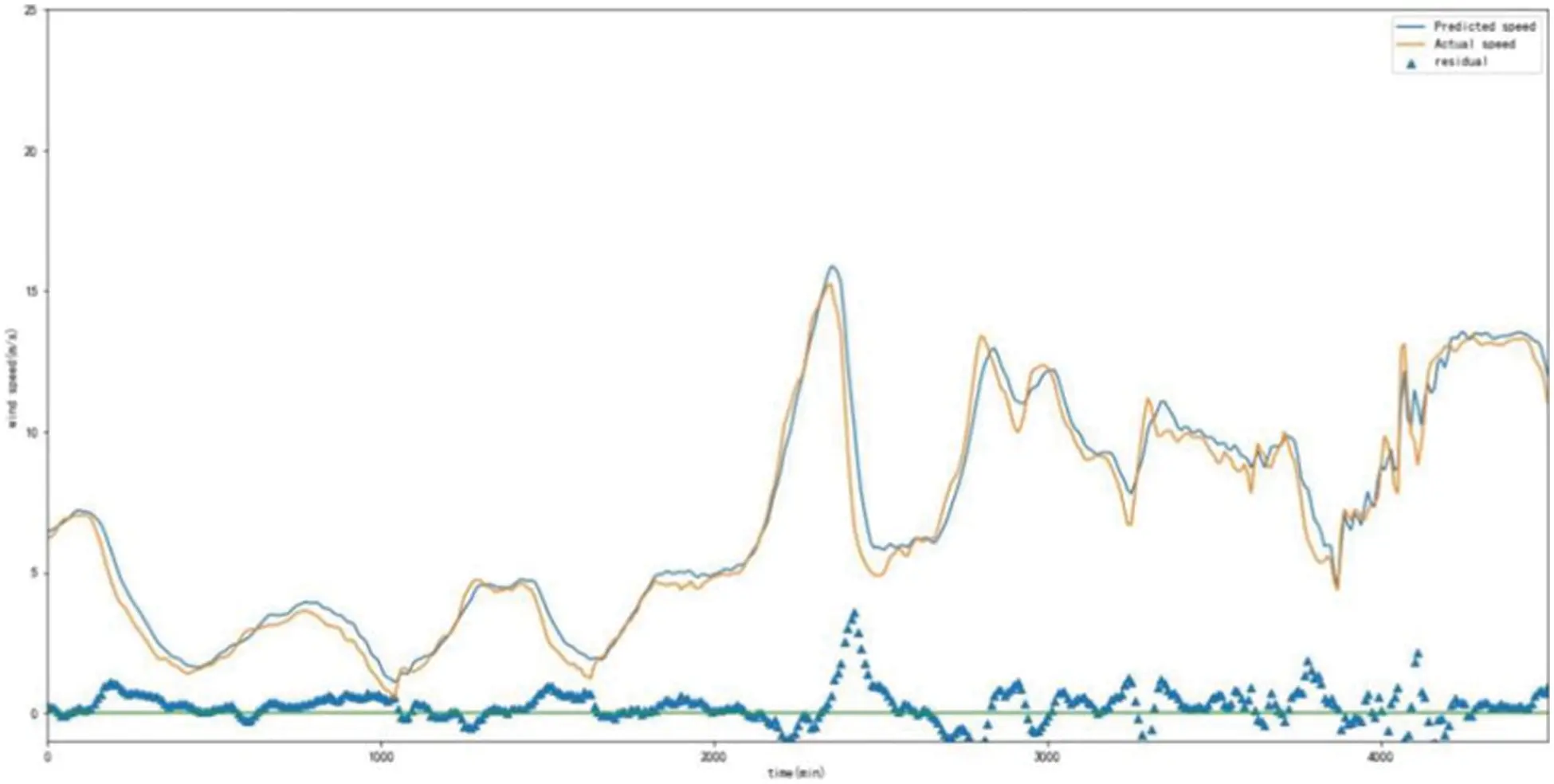Figure 8: Partial wind speed prediction results of VCGA model at 20-min intervals

From Fig. 8, it is evident that the VCGA model has a very high fitting degree and can accurately reflect the trend of the actual value. The residual analysis results illustrate that the prediction residual of the model is uniform and randomly distributed on both sides of the zero baselines, indicating that there is no systematic error in the modeling process . Therefore, the model is feasible for short-term wind speed prediction.

For better intuitive inspecting, the differences between VCGA and other algorithms, Fig. 9 is plotted to show different models’ wind speed prediction results when the prediction time is 20 min. These algorithms include VCGA, LSTM, GRU, CNN-GRU, VMD-CNN-GRU, and CNN-GRU-Attention. Fig. 9 also shows the good predictive performance of the VCGA model, where it outperformed these algorithms in terms of the highest fitting degree.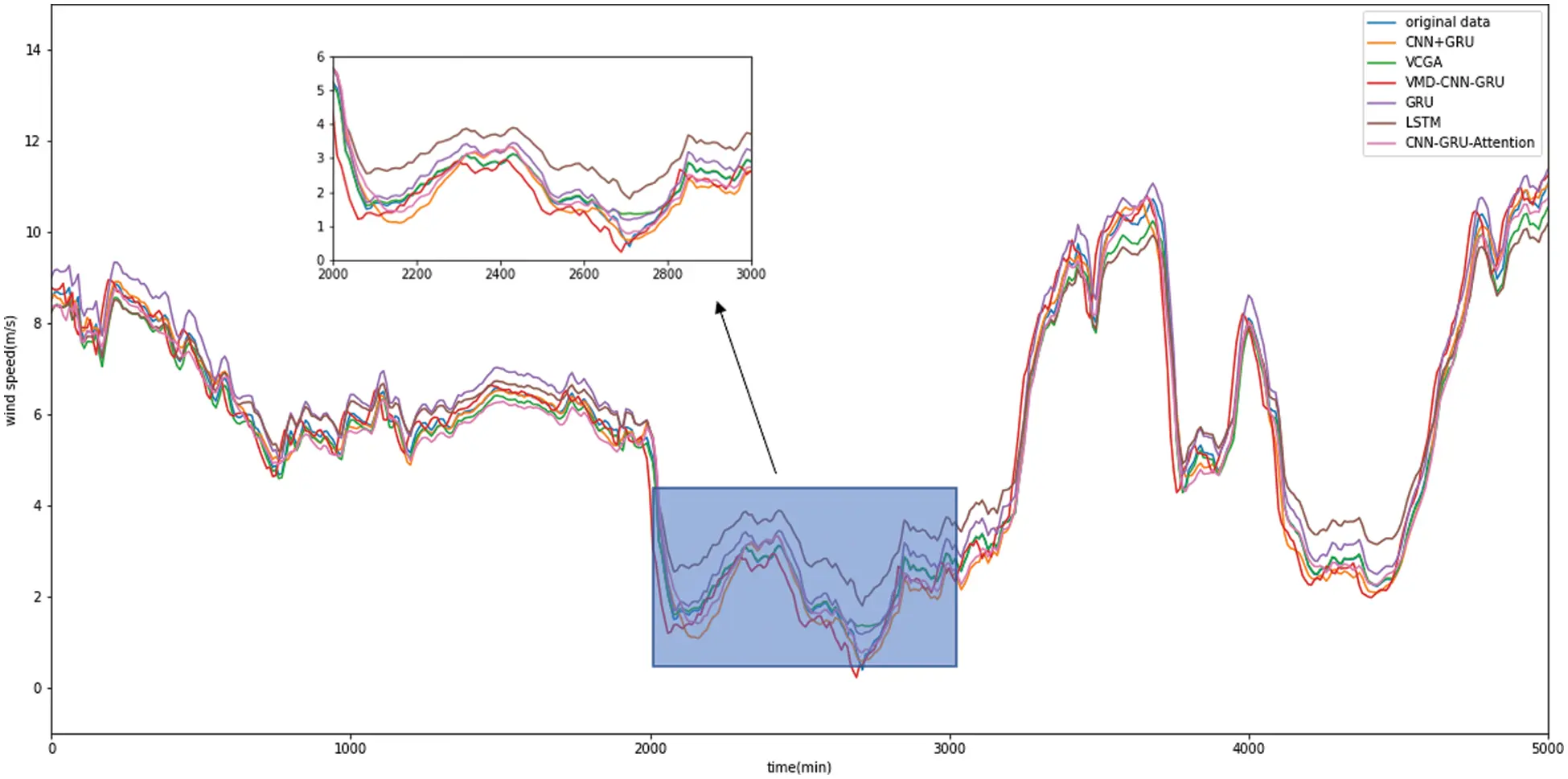Figure 9: Wind speed prediction results of different models at 20-min intervals

5  Conclusion

This paper proposes a VCGA algorithm for short-term wind speed prediction. This algorithm uses VMD to stabilize the wind speed series to obtain IMF components. The included attention mechanism aims to reduce the computational burden and better extract features. The SENet layer extracts the spatial characteristics from IMF components. Then, the GRU network connected with the attention mechanism is used to extract time-domain features. Finally, wind speed predictions are obtained after merging spatial and time-domain features.

The simulation results show that VCGA can fully explore the spatio-temporal characteristics of wind speed series and effectively improve the accuracy of short-term wind speed prediction. It also has vast application potential. However, more factors that may affect wind prediction, such as temperature, humidity, and altitude, will be considered in future studies. Thus, the model developed here will be modified to obtain more accurate prediction results. In addition, we will also research how to introduce more excellent algorithms, such as BiGRU, into the model for adapting to the wind speed prediction environment and improving forecasting performance.

Acknowledgement: This paper is supported by the undergraduate training program for innovation and entrepreneurship of NUIST (XJDC202110300239).

Funding Statement: This paper is supported by the undergraduate training program for innovation and entrepreneurship of NUIST (XJDC202110300239).

Conflicts of Interest: The authors declare that they have no conflicts of interest to report regarding the present study.

## References

1. G. Herbert, S. Iniyan, E. Sreevalsan and S. Rajapandian, “A review of wind energy technologies,” Renewable & Sustainable Energy Reviews, vol. 11, no. 6, pp. 1117–1145, 2007.
2. X. Mi, H. Liu and Y. Li, “Wind speed prediction model using singular spectrum analysis, empirical mode decomposition and convolutional support vector machine,” Energy Conversion and Management, vol. 180, pp. 196–205, 2019.
3. K. G. Sheela and S. N. Deepa, “Neural network-based hybrid computing model for wind speed prediction,” Neurocomputing, vol. 122, pp. 425–429, 201
4. A. J. Simmons and A. Hollingsworth, “Some aspects of the improvement in skill of numerical weather prediction,” Quarterly Journal of the Royal Meteorological Society, vol. 128, no. 580, pp. 647–677, 2002.
5. R. J. Abrahart and L. See, “Comparing neural network and autoregressive moving average techniques for the provision of continuous river flow forecasts in two contrasting catchments,” Hydrological Processes, vol. 14, no. 11, pp. 2157–2172, 201
6. S. Hua and Z. Sun, “Support vector machine approach for protein subcellular localization prediction,” Bioinformatics, vol. 17, no. 8, pp. 721–728, 2001.
7. G. B. Huang, Q. Y. Zhu and C. K. Siew, “Extreme learning machine: a new learning scheme of feedforward neural networks,” in 2004 IEEE int. joint conf. on neural networks (IEEE Cat. No. 04CH37541), Budapest, Hungary, pp. 985–990, 2005.
8. X. X. Zhu, R. Z. Liu, Y. Chen, X. X. Gao, Y. Wang et al., “Wind speed behaviors feather analysis and its utilization on wind speed prediction using 3D-CNN,” Energy, vol. 236, pp. 121523, 2021.
9. X. R. Zhang, X. Sun, W. Sun, T. Xu and P. P. Wang, “Deformation expression of soft tissue based on BP neural network,” Intelligent Automation & Soft Computing, vol. 32, no. 2, pp. 1041–1053, 2022.
10. I. Tanaka and H. Ohmori, “Method selection in different regions for short-term wind speed prediction in Japan,” in 2015 54th Annual Conf. of the Society of Instrument and Control Engineers of Japan (SICE), Hangzhou, China, pp. 189–194, 2015.
11. C. Li, G. Tang, X. Xue, A. Saeed and X. Hu, “Short-Term Wind Speed Interval Prediction Based on Ensemble GRU Model,” IEEE Transactions on Sustainable Energy, vol. 11, no. 3, pp. 1370–1380, 2019.
12. G. G. Chen, B. R. Tang, X. J. Zeng, P. Zhou, P. Kang et al., “Short-term wind speed forecasting based on long short-term memory and improved BP neural network,” International Journal of Electrical Power & Energy Systems, vol. 134, pp. 107365, 2022.
13. K. Dragomiretskiy and D. Zosso, “Variational mode decomposition,” IEEE Transactions on Signal Processing, vol. 62, no. 3, pp. 531–544, 2014.
14. M. Bekara and M. V. D. Baan, “Random and coherent noise attenuation by empirical mode decomposition,” Geophysics, vol. 74, no. 5, pp. 89–98, 2009.
15. S. Wang, N. Zhang, L. Wu and Y. Wang, “Wind speed forecasting based on the hybrid ensemble empirical mode decomposition and GA-BP neural network method,” Renewable Energy, vol. 94, pp. 629–636, 2016.
16. S. Song, C. Lan, J. Xing, W. Zeng and J. Liu, “Spatio-temporal attention-based LSTM networks for 3D action recognition and detection,” IEEE Transactions on Image Processing, vol. 27, no. 7, pp. 3459–3471, 2018.
17. D. R. Liu, S. J. Lee, Y. Huang and C. J. Chiu, “Air pollution forecasting based on attention-based LSTM neural network and ensemble learning,” Expert Systems, vol. 37, no. 3, pp. 12511, 2020.
18. J. R. Zhang, F. A. Liu, W. Z. Xu and H. Yu, “Feature fusion text classification model combining CNN and BiGRU with multi-attention mechanism,” Future Internet, vol. 11, no. 11, pp. 237–249, 2019.
19. A. M. Almars, “Attention-based bi-lstm model for arabic depression classification,” Computers,” Materials & Continua, vol. 71, no. 2, pp. 3091–3106, 2022.
20. Q. Zhu, J. Chen, D. Shi, L. Zhu, X. Bai et al., “Learning temporal and spatial correlations jointly: A unified framework for wind speed prediction,” IEEE Transactions on Sustainable Energy, vol. 11, no. 1, pp. 509–523, 2019.
21. G. Zhang and R. Heusdens, “Bi-alternating direction method of multipliers,” in IEEE Int. Conf. on Acoustics, Speech and Signal Processing, Vancouver, BC, Canada, pp. 3317–3321, 2013.
22. P. H. Kuo and C. J. Huang, “A high precision artificial neural networks model for short-term energy load forecasting,” Energies, vol. 11, no. 1, pp. 213, 2018.
23. J. H. Zhu, J. H. Pei and Z. Yang, “Research on convolution kernel initialization method in convolutional neural network (CNN) training,” Journal of Signal Processing, vol. 35, no. 4, pp. 641–648, 2019.
24. S. Hochreiter and J. Schmidhuber, “Long short-term memory,” Neural Computation, vol. 9, no. 8, pp. 1735–1780, 1997.
25. W. Fang, Y. P. Chen and Q. Y. Xue, “Survey on research of RNN-based spatio-temporal sequence prediction algorithms,” Journal on Big Data, vol. 3, no. 3, pp. 97–110, 2021.
26. F. Rui, Z. Zuo and L. Li. “Using LSTM and GRU neural network methods for traffic flow prediction,” in 2016 31st Youth Academic Annual Conf. of Chinese Association of Automation (YAC) IEEE, Wuhan, China, 2016.
27. D. Bahdanau, K. Cho and Y. Bengio, “Neural machine translation by jointly learning to align and translate,” Computer Science, vol. 7, pp. 1409.0473, 2014.
28. M. T. Luong, H. Pham and C. D. Manning, “Effective approaches to attention-based neural machine translation,” Computer Science, vol. 5, pp. 1508.04025, 2015.
29. J. Qian, M. Zhu, Y. Zhao and X. He, “Short-term wind speed prediction with a two-layer attention-based lstm,” Computer Systems Science and Engineering, vol. 39, no. 2, pp. 197–209, 2021.
30. X. Ran, Z. Shan, Y. Fan and C. Lin, “An LSTM-based method with attention mechanism for travel time prediction,” Sensors, vol. 19, no. 4, pp. 861, 2019.
31. Y. Zhu, W. Sun, X. Cao, C. Wang, D. Wu et al., “TA-CNN: Two-way attention models in deep convolutional neural network for plant recognition,” Neurocomputing, vol. 365, pp. 191–200, 2019.
32. Y. S. Xue, N. Chen and S. M. Wang, “A review on wind speed prediction using spatial correlation,” Automation of Electric Power Systems, vol. 41, no. 10, pp. 161–169, 2017.
33. X. R. Zhang, W. F. Zhang, W. Sun, X. M. Sun and S. K. Jha, “A robust 3-D medical watermarking based on wavelet transform for data protection,” Computer Systems Science & Engineering, vol. 41, no. 3, pp. 1043–1056, 2022.
34. J. Hu, L. Shen, G. Sun S. Albanie and E. Wu, “Squeeze-and-excitation networks,” IEEE Transactions on Pattern Analysis and Machine Intelligence, vol. 42, no. 8, pp. 2011–2023, 2019.
35. N. Srivastava, G. Hinton, A. Krizhevsky, I. Sutskever and R. Salakhutdinov, “Dropout: A simple Way to prevent neural networks from overfitting,” Journal of Machine Learning Research, vol. 15, no. 1, pp. 1929–1958, 2014.
36. D. Kingma and J. Ba, “Adam: A method for stochastic optimization,” Computer Science, vol. 9, pp. 1412.6980, 2014.
37. J. Nevitt and G. R. Hancock, “Improving the root mean square error of approximation for nonnormal conditions in structural equation modeling,” Journal of Experimental Education, vol. 68, no. 3, pp. 251–268, 2000.
38. A. D. Myttenaere, B. Golden, B. L. Grand and F. Rossi, “Using the mean absolute percentage error for regression models,” Neurocomputing, vol. 1, pp. 1506.04176, 2015.
39. A. Ding, Y. Zhang, L. Zhu, H. Li and L. Huang, “Intelligent recognition of rough handling of express parcels based on cnn-gru with the channel attention mechanism,” Journal of Ambient Intelligence and Humanized Computing, vol. 12, no. 6, pp. 1–18, 2021.
40. A. Graves, A. R. Mohamed and G. Hinton, “Speech recognition with deep recurrent neural networks,” in 2013 IEEE Int. Conf. on Acoustics, Speech and Signal Processing, Vancouver, BC, Canada, pp. 6645–6649, 2013.
41. K. L. Du and M. Swamy, “Neural networks and statistical learning,” Berlin, Germany: Springer Publishing Company, Incorporated, 2013. [Online]. Available: https://link.springer.com/book/10.1007/978-1-4471-5571-3.
42. K. He, X. Zhang, S. Ren and J. Sun, “Deep residual learning for image recognition,” in Proc. of the IEEE Conf. on Computer Vision and Pattern Recognition, Las Vegas, NV, USA, pp. 770–778, 2016.This work is licensed under a Creative Commons Attribution 4.0 International License, which permits unrestricted use, distribution, and reproduction in any medium, provided the original work is properly cited.